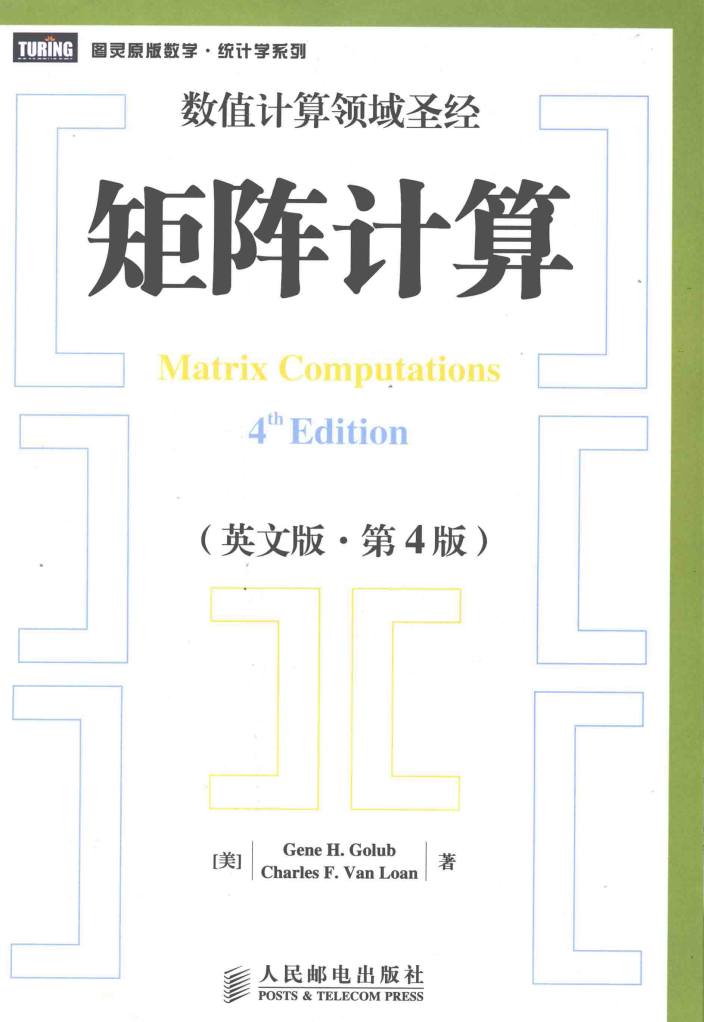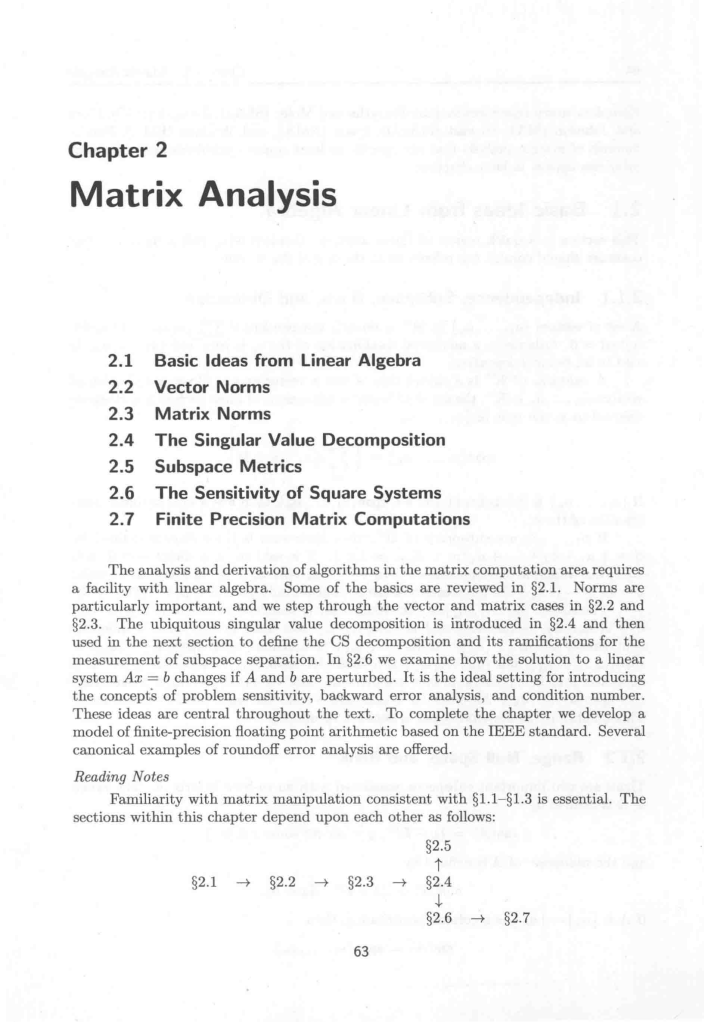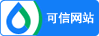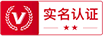### 注册

•• 获取手机验证码 60
• 注册

### 找回密码

•• 获取手机验证码60
• 找回

### 矩阵分析外文翻译资料

2023-03-16 11:03

2.1 线性代数的基本思想

2.1.1 线性无关，子空间，基，维数

.

2.1.2 值域，零空间，秩

.

A的零空间定义为

.

.

.

.

2.1.3 逆矩阵

2.1.4 Sherman-Morrison-Woodbury公式

2.1.5 正交性

2.1.6 行列式

.此外，当且仅当A是非奇异的。

2.1.7 特征值和特征向量

2.1.8 微分

2.2 向量范数

2.2.1 定义

2.2.2 向量范数的性质

2.2.3 绝对误差和相对误差

2.2.4 收敛性

,

The analysis and derivation of algorithms in the matrix computation area requires a facility with linear algebra. Some of the basics are reviewed in sect;2.1. Norms are particularly important, and we step through the vector and matrix cases in sect;2.2 and sect;2.3. The ubiquitous singular value decomposition is introduced in sect;2.4 and then used in the next section to define the CS decomposition and its ramifications for the measurement of subspace separation. In sect;2.6 we examine how the solution to a linear system changes if A and b are perturbed. It is the ideal setting for introducing the concepts of problem sensitivity, backward error analysis, and condition number. These ideas are central throughout the text. To complete the chapter we develop a model of finite-precision floating point arithmetic based on the IEEE standard. Several canonical examples of roundoff error analysis are offered.

2.1 Basic Ideas from Linear Algebra

This section is a quick review of linear algebra. Readers who wish a more detailed coverage should consult the references at the end of the section.

2.1.1 Independence, Subspace, Basis, and Dimension

A set of vectors in is linearly independent if implies . Otherwise, a nontrivial combination of the is zero and is said to be linearly dependent.

A subspace of is a subset that is also a vector space. Given a collection of vectors, the set of all linear combinations of these vectors is a subspace referred to as the span of ：

.

If is a independent and , then b is a unique linear combination of the .

If are subspaces of , then their sum is the subspace defined by .S is said to be a direct sum if each has a unique representation with . In this case we write . The intersection of the is also a subspace, .

The subset is a maximal linearly independent subset of if it is linearly independent and is not properly contained in any linearly independent subset of . If is maximal, then and is a basis for span. If is a subspace, then it is possible to find independent basic vectors such that . All bases for a subspace S have the same number of elements. This number is the dimension and is denoted by .

2.1.2 Range, Null Space, and Rank

There are two important subspaces associated with an m-by-n matrix A. The range of A is defined by

.

And the of A is defined by

.

If is a column partitioning, then

.

The rank of a matrix A is defined by

.

If , then

.

We say that is rank deficient if . The rank of a matrix is the maximal### 最新文档•